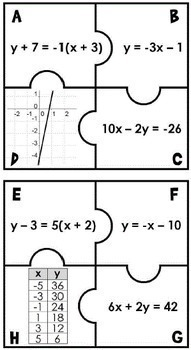DID YOU KNOW:
Seamlessly assign resources as digital activities

Learn how in 5 minutes with a tutorial resource. Try it Now

Learn More# Linear Equations Puzzle - print and digital8th - 9th
Subjects
Standards
Resource Type
Formats Included
• PDF
•Google Apps™
Pages
7 pagesThe Teacher-Author indicated this resource includes assets from Google Workspace (e.g. docs, slides, etc.).

#### Also included in

1. This bundle includes 5 algebra puzzles covering slope, linear equations, inverse functions and quadratics. You can read more about each puzzle in the individual descriptions below:Slope Puzzle - print and digitalStudents cut out 24 puzzle pieces with different representations of slope (graphs, table
\$14.50
\$17.00
Save \$2.50

### Description

*Includes both printable and digital in Google Slides.

In this linear equations activity, match 24 puzzle pieces with 3 different representations of the same equation - slope-intercept form, point-slope form, and standard form. The 4th piece of each puzzle shows a graph or table of the equation. Students fit same-equation pieces together. When completed, each student or pair of students will make 6 puzzles, each with 4 pieces.

This activity is great practice for translating between the different forms of linear equations and also for recognizing an equation given its graph or table.

Now also included is a digital GOOGLE Slides version for students working online. Students match the same puzzle pieces, just on their screen, then share it back with you. The digital version spans 2 slides so that students do not have to move any pieces from one slide to another. Students will complete 3 puzzles on each slide.

Puzzle pieces are not matched on the sheets so that students can be in charge of cutting them out. The preview is a completed activity printed on colorful paper (actual puzzle pieces are larger, 2 completed puzzles will fit on 1 piece of paper). Includes both print + interactive digital versions

Included in: Algebra Activities Bundle w/ digital updates

>> See more print + digital math activities here.

You may also like:

Linear Equations Flippables - print and digital

Graphing Linear Equations Digital Math Escape Room

Algebra Word Wall - print and digital

Total Pages
7 pages
Included
Teaching Duration
N/A
Report this Resource to TpT
Reported resources will be reviewed by our team. Report this resource to let us know if this resource violates TpT’s content guidelines.

### Standards

to see state-specific standards (only available in the US).
Write a function that describes a relationship between two quantities.
Understand that the graph of an equation in two variables is the set of all its solutions plotted in the coordinate plane, often forming a curve (which could be a line).
Construct a function to model a linear relationship between two quantities. Determine the rate of change and initial value of the function from a description of a relationship or from two (𝘹, 𝘺) values, including reading these from a table or from a graph. Interpret the rate of change and initial value of a linear function in terms of the situation it models, and in terms of its graph or a table of values.